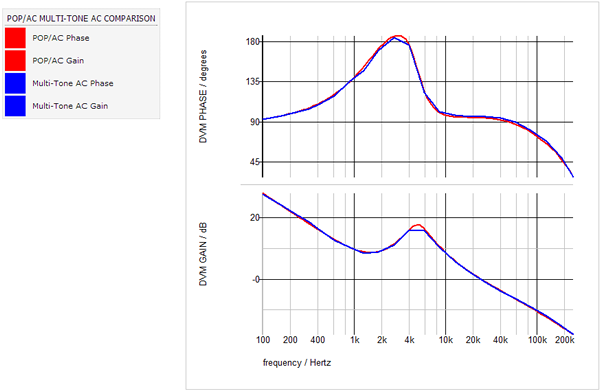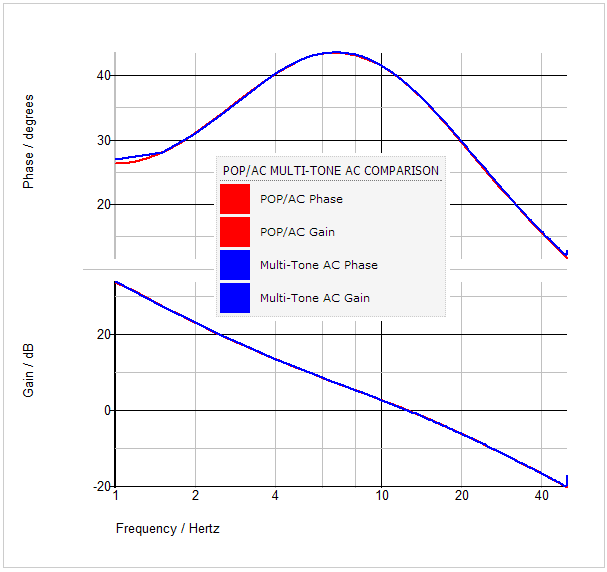DVM - Design Verification Module

# Multi-Tone AC Analysis - Introduction

The SIMPLIS Periodic Operating Point (POP) analysis followed by an AC analysis has a proven record of quickly and accurately measuring the AC loop response of switching power supplies. This analysis works well for stable systems where the feedback signals are represented by double precision numbers. Two distinct classes of converters exist which are incompatible with the SIMPLIS POP analysis:

• Digitally controlled converters in which the feedback variables are quantized into discrete levels.
• Converters with multiple, asynchronous frequencies, such as a PFC converter.

The Multi-Tone AC Analysis was designed for these types of converters.

In this Topic Hide

## Characterizing Digital Control Loops

In digitally controlled systems where the signals in the feedback loop are quantized into discrete levels, the POP/AC analysis is typically not applicable. The extremely small perturbation of the injected signal during the AC analysis is not large enough to produce a control-loop response. The Multi-Tone AC analysis, now available in the Design Verification Module (DVM) in SIMetrix/SIMPLIS 7.1, is able to provide an AC analysis of such digitally controlled systems, including closed-loop response, input and output impedance, as well as conducted susceptibility. Below is a comparison of closed-loop gain and phase results from a digitally controlled DC/DC converter.The red curves represent the POP/AC data where the PID control signals are modeled as double precision numbers. The blue curves are generated by the new Multi-Tone AC analysis where the quantized finite bit-width of the PID feedback signals are modeled. In both cases, the PID coefficients and the power stage components are identical.

## Characterizing PFC Control Loops

For PFC converters using an AC input and variable frequency control, the POP/AC analysis is not appropriate. In these cases, the Multi-Tone AC analysis offers a way to characterize virtually any control loop. Below are the loop gain and phase results from a single-phase, continuous-conduction mode PFC converter.The red curves represent the POP/AC data using a PWM multiplier; the blue curves show the Multi-Tone AC results using the full NLB multiplier.

The Multi-Tone AC analysis injects multiple frequencies into the circuit under test during a transient simulation and then calculates the AC results using a Fourier analysis. The signal amplitude is set by the user to be large enough to insure the control loop is perturbed and that there will be a valid AC response to the perturbation. Because Multi-Tone AC analysis uses a transient simulation, the technique can be applied to any converter. POP convergence is not a requirement.

Both linear and decade frequency sweep modes are available with the four Multi-Tone tests objectives that are built in to DVM:

• Bode Plot
• Output Impedance
• Input Impedance
• Conducted Susceptibility.

Using the built-in testplans for DC/DC and AC/DC converters, you can, in five minutes or less, convert a working design to run the Multi-Tone AC analysis.

For more details, see Multi-Tone AC Analysis Theory.